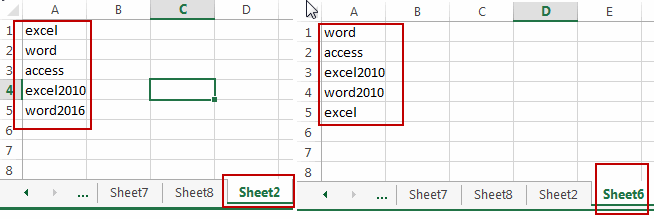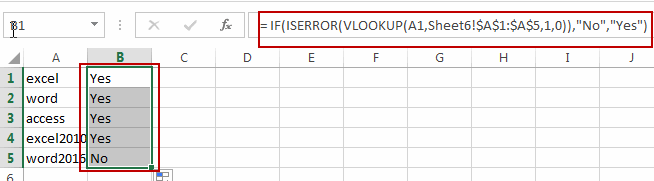# How to Compare Two Lists in Different Worksheets using VLOOKUP in Excel

This post will guide you how to compare two lists in different worksheets in Excel. How do I compare two lists in different worksheets with VLOOKUP function in Excel 2013/2016.

## Comparing Two Lists in Different Worksheets

Assuming that you have two lists of data in the different worksheets, and you want to compare those two lists and try to check the values in the first list(Sheet2 – A1:A5) if it is in another list (Sheet6 – A1:A5). How to do it. You can use an Excel formula based on the VLOOKUP function, the IF function and the ISERROR function.Here is the formula that comparing two lists in different worksheets:

`= IF(ISERROR(VLOOKUP(A1,'Sheet6'!\$A\$1:\$A\$5,1,0)),"No","Yes")`

You need to type this formula into cell B1 in the first worksheet that containing the first list data. And then drag the Fill Handle down to the cells to comparing other values with the second list in another worksheet.You would notice that the checking results have been returned in column B. if it returns Yes, it indicates that this cell value is also in the second list in another worksheet. If it return No, it indicates that this cell value is not in the second list.

### Related Functions

• Excel IF function
The Excel IF function perform a logical test to return one value if the condition is TRUE and return another value if the condition is FALSE. The IF function is a build-in function in Microsoft Excel and it is categorized as a Logical Function.The syntax of the IF function is as below:= IF (condition, [true_value], [false_value])….
• Excel VLOOKUP function
The Excel VLOOKUP function lookup a value in the first column of the table and return the value in the same row based on index_num position.The syntax of the VLOOKUP function is as below:= VLOOKUP (lookup_value, table_array, column_index_num,[range_lookup])….
• Excel IFERROR function
The Excel IFERROR function returns an alternate value you specify if a formula results in an error, or returns the result of the formula.The syntax of the IFERROR function is as below:= IFERROR (value, value_if_error)….